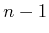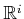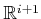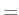#### Semi-algebraic projections are semi-algebraic

The following is implied by the Tarski-Seidenberg Theorem :

A projection of a semi-algebraic set from dimensionto dimensionis a semi-algebraic set.

This gives a kind of closure of semi-algebraic sets under projection, which is required to ensure that every projection of a semi-algebraic set inleads to a semi-algebraic set in. This property is actually not true for (real) algebraic varieties, which were introduced in Section 4.4.1. Varieties are defined using only therelation and are not closed under the projection operation. Therefore, it is a good thing (not just a coincidence!) that we are using semi-algebraic sets.

Steven M LaValle 2020-08-14